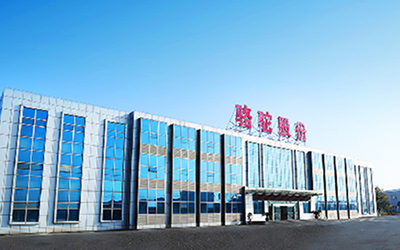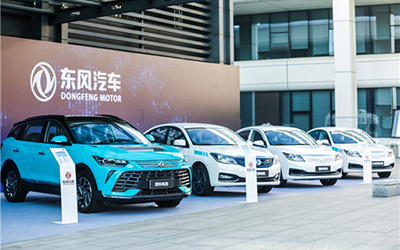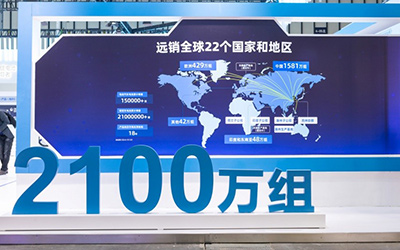# 合作双赢 共谋发展 福田戴姆勒一行赴骆驼股份考察交流# 纵深推进“双创” 赋能东风高质量发展# 星恒年度大秀南京献映 全维升级定义“技领全球”# 当新中产手握30W 他们会选什么座驾？

30万左右可以选择的SUV车型非常多，新中产到底会如何选择呢？他们不盲目的品牌追随者，更加注重产品的本质，同时讲究格调、有内涵。

# 布雷博全新智能制动系统SENSIFY重磅发布

2021年10月25日，布雷博重磅推出SENSIFY制动系统，布雷博SENSIFY制动系统预计将于2024年初上市。

# 年轻智能风范 MG ONE实力秒杀长安UNI-T

MGONE与长安UNI-T在性能与人群定位方面有着很多相似的地方，这两款产品哪款更胜一筹呢？

12345 下5页 下一页 尾页 共 2480 页

﻿
• 快速找车
• 选择品牌
• 选择品牌
• A  奥迪
• A  阿斯顿·马丁
• A  阿尔法·罗密欧
• B  宝沃
• B  布加迪
• B  巴博斯
• B  保时捷
• B  宾利
• B  奔驰
• B  宝马
• B  本田
• B  别克
• B  标致
• B  比亚迪
• B  宝骏
• B  北汽制造
• B  北汽新能源
• B  北汽幻速
• B  北汽威旺
• B  北京汽车
• B  奔腾
• B  北汽绅宝
• B  北汽昌河
• C  长安欧尚
• C  长安
• C  长安凯程
• C  长城
• D  大众
• D  道奇
• D  DS
• D  东南
• D  东风风神
• D  东风风行
• D  东风小康
• D  东风风度
• D  东风
• F  福特
• F  丰田
• F  菲亚特
• F  法拉利
• F  福田
• F  福迪
• F  福汽启腾
• G  观致
• G  广汽传祺
• G  广汽吉奥
• G  GMC
• H  红旗
• H  汉腾汽车
• H  哈弗
• H  哈飞
• H  海格
• H  海马
• H  华颂
• H  黄海
• H  华泰
• H  恒天
• J  捷途
• J  几何汽车
• J  捷达
• J  吉利汽车
• J  捷豹
• J  Jeep
• J  江淮
• J  江铃
• J  金杯
• J  九龙
• J  金旅
• K  凯翼
• K  凯迪拉克
• K  克莱斯勒
• K  科尼塞克
• K  卡威
• K  开瑞
• L  路虎
• L  林肯
• L  劳斯莱斯
• L  兰博基尼
• L  雷克萨斯
• L  铃木
• L  领克
• L  雷诺
• L  理念
• L  力帆
• L  莲花汽车
• L  猎豹
• L  路特斯
• L  陆风
• M  马自达
• M  MG
• M  MINI
• M  玛莎拉蒂
• M  摩根
• M  迈凯轮
• N  纳智捷
• O  欧拉
• O  欧宝
• O  讴歌
• O  欧朗
• Q  奇瑞
• Q  起亚
• Q  启辰
• R  日产
• R  荣威
• R  瑞麒
• S  SERES赛力斯
• S  三菱
• S  斯威汽车
• S  萨博
• S  smart
• S  斯柯达
• S  斯巴鲁
• S  思铭
• S  双龙
• S  上汽大通
• S  双环
• T  特斯拉
• T  腾势
• W  蔚来
• W  沃尔沃
• W  WEY
• W  五菱汽车
• W  五十铃
• W  威兹曼
• W  威麟
• X  现代
• X  雪佛兰
• X  星途
• X  雪铁龙
• X  小鹏汽车
• X  西雅特
• Y  一汽
• Y  英菲尼迪
• Y  英致
• Y  依维柯
• Y  野马汽车
• Y  永源
• Z  众泰
• Z  中华
• Z  中兴
• Z  知豆
• 选择车系
• 选择车系
• 车型对比
• 选择品牌
• 选择品牌
• A  奥迪
• A  阿斯顿·马丁
• A  阿尔法·罗密欧
• B  宝沃
• B  布加迪
• B  巴博斯
• B  保时捷
• B  宾利
• B  奔驰
• B  宝马
• B  本田
• B  别克
• B  标致
• B  比亚迪
• B  宝骏
• B  北汽制造
• B  北汽新能源
• B  北汽幻速
• B  北汽威旺
• B  北京汽车
• B  奔腾
• B  北汽绅宝
• B  北汽昌河
• C  长安欧尚
• C  长安
• C  长安凯程
• C  长城
• D  大众
• D  道奇
• D  DS
• D  东南
• D  东风风神
• D  东风风行
• D  东风小康
• D  东风风度
• D  东风
• F  福特
• F  丰田
• F  菲亚特
• F  法拉利
• F  福田
• F  福迪
• F  福汽启腾
• G  观致
• G  广汽传祺
• G  广汽吉奥
• G  GMC
• H  红旗
• H  汉腾汽车
• H  哈弗
• H  哈飞
• H  海格
• H  海马
• H  华颂
• H  黄海
• H  华泰
• H  恒天
• J  捷途
• J  几何汽车
• J  捷达
• J  吉利汽车
• J  捷豹
• J  Jeep
• J  江淮
• J  江铃
• J  金杯
• J  九龙
• J  金旅
• K  凯翼
• K  凯迪拉克
• K  克莱斯勒
• K  科尼塞克
• K  卡威
• K  开瑞
• L  路虎
• L  林肯
• L  劳斯莱斯
• L  兰博基尼
• L  雷克萨斯
• L  铃木
• L  领克
• L  雷诺
• L  理念
• L  力帆
• L  莲花汽车
• L  猎豹
• L  路特斯
• L  陆风
• M  马自达
• M  MG
• M  MINI
• M  玛莎拉蒂
• M  摩根
• M  迈凯轮
• N  纳智捷
• O  欧拉
• O  欧宝
• O  讴歌
• O  欧朗
• Q  奇瑞
• Q  起亚
• Q  启辰
• R  日产
• R  荣威
• R  瑞麒
• S  SERES赛力斯
• S  三菱
• S  斯威汽车
• S  萨博
• S  smart
• S  斯柯达
• S  斯巴鲁
• S  思铭
• S  双龙
• S  上汽大通
• S  双环
• T  特斯拉
• T  腾势
• W  蔚来
• W  沃尔沃
• W  WEY
• W  五菱汽车
• W  五十铃
• W  威兹曼
• W  威麟
• X  现代
• X  雪佛兰
• X  星途
• X  雪铁龙
• X  小鹏汽车
• X  西雅特
• Y  一汽
• Y  英菲尼迪
• Y  英致
• Y  依维柯
• Y  野马汽车
• Y  永源
• Z  众泰
• Z  中华
• Z  中兴
• Z  知豆
• 选择车系
• 选择车系
• 选择车型
• 选择车型
• 意见反馈